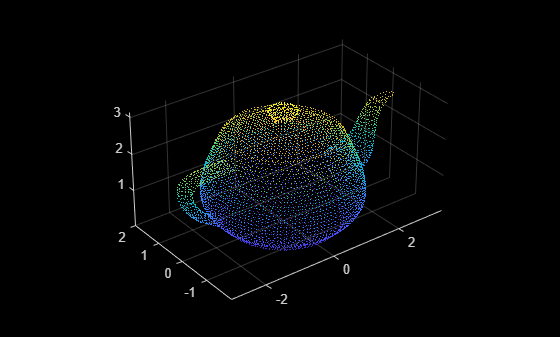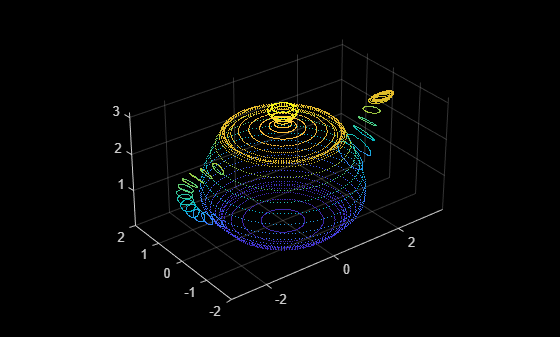# pcdownsample

Downsample a 3-D point cloud

## Syntax

``ptCloudOut = pcdownsample(ptCloudIn,'random',percentage)``
``ptCloudOut = pcdownsample(ptCloudIn,'gridAverage',gridStep)``
``ptCloudOut = pcdownsample(ptCloudIn,'nonuniformGridSample',maxNumPoints)``
``[ptCloudOut,indices] = pcdownsample(___)``
``___ = pcdownsample(___,Name=Value)``

## Description

````ptCloudOut = pcdownsample(ptCloudIn,'random',percentage)` returns a downsampled point cloud with random sampling and without replacement. The `percentage` input specifies the portion of the input to return to the output.```

example

````ptCloudOut = pcdownsample(ptCloudIn,'gridAverage',gridStep)` returns a downsampled point cloud using a box grid filter. The `gridStep` input specifies the size of a 3-D box.```
````ptCloudOut = pcdownsample(ptCloudIn,'nonuniformGridSample',maxNumPoints)` returns a downsampled point cloud using nonuniform box grid filter. You must set the maximum number of points in the grid box, `maxNumPoints`, to at least `6`.```
````[ptCloudOut,indices] = pcdownsample(___)` returns the linear indices for the points that are included in the downsampled point cloud. This syntax applies only when you use the `'random'` or `'nonuniformGridSample'` downsampling methods.```
````___ = pcdownsample(___,Name=Value)` specifies a name-value argument in addition to any combination of arguments from previous syntaxes.```

## Examples

collapse all

`ptCloud = pcread('teapot.ply');`

Set the 3-D resolution to be (0.1 x 0.1 x 0.1).

```gridStep = 0.1; ptCloudA = pcdownsample(ptCloud,'gridAverage',gridStep);```

Visualize the downsampled data.

```figure; pcshow(ptCloudA);```Compare the point cloud to data that is downsampled using a fixed step size.

```stepSize = floor(ptCloud.Count/ptCloudA.Count); indices = 1:stepSize:ptCloud.Count; ptCloudB = select(ptCloud, indices); figure; pcshow(ptCloudB);```Create a point cloud with all points sharing the same coordinates.

`ptCloud = pointCloud(ones(100,3));`

Set the 3-D resolution to a small value.

`gridStep = 0.01;`

The output now contains only one unique point.

`ptCloudOut = pcdownsample(ptCloud,'gridAverage',gridStep)`
```ptCloudOut = pointCloud with properties: Location: [1 1 1] Count: 1 XLimits: [1 1] YLimits: [1 1] ZLimits: [1 1] Color: [0x3 uint8] Normal: [0x3 double] Intensity: [0x1 double] ```

Load an organized point cloud from a saved MAT-file.

```ld = load('drivingLidarPoints.mat'); orgPtCloud = ld.ptCloud;```

Downsample the point cloud.

```percentage = 0.1; orgPtCloudOut = pcdownsample(orgPtCloud,'random',percentage,PreserveStructure=true);```

## Input Arguments

collapse all

Point cloud, specified as a `pointCloud` object.

Random downsample method, specified as `'random'`. The `'random'` method is more efficient than the `'gridAverage'` downsample method, especially when it is applied before point cloud registration.

Percentage of input, specified as a nonnegative scalar in the range [0, 1]. The `percentage` input specifies the portion of the input for the function to return.

Grid average downsample method, specified as `'gridAverage'`. Points within the same box are merged to a single point in the output. Their color and normal properties are averaged accordingly. This method preserves the shape of the point cloud better than the `'random'` downsample method.

The function computes the axis-aligned bounding box for the entire point cloud. The bounding box is divided into grid boxes of size specified by `gridStep`. Points within each grid box are merged by averaging their locations, colors, and normals.

Size of 3-D box for grid filter, specified as a numeric value. Increase the size of `gridStep` when there are not enough resources to construct a large fine-grained grid.

Data Types: `single` | `double`

Nonuniform grid sample method, specified as `'nonuniformGridSample'`. When you use the `'nonuniformGridSample'` algorithm, the normals are computed on the original data prior to downsampling. The downsampled output preserves more accurate normals.

Maximum number of points in grid box, specified as an integer greater than `6`. The method randomly selects a single point from each box. If the normal was not provided in the input point cloud, this method automatically fills in the normal property in the `ptCloudOut` output.

### Name-Value Arguments

Specify optional pairs of arguments as `Name1=Value1,...,NameN=ValueN`, where `Name` is the argument name and `Value` is the corresponding value. Name-value arguments must appear after other arguments, but the order of the pairs does not matter.

Before R2021a, use commas to separate each name and value, and enclose `Name` in quotes.

Example: `PreserveStructure=true` preserves the organized structure of a point cloud.

Preserve the organized structure of a point cloud, specified as `true` or `false`. The table describes the point cloud structure according to the value of `PreserveStructure`.

`PreserveStructure`The function returns
`true`

An organized, downsampled, point cloud.

The Location property that describes the structure of the point cloud, contains an M-by-N-by-3 matrix.

Points that are not selected in the downsampled point cloud are filled with `NaN`, and the corresponding color is set to `[0 0 0]`.

To return an organized point cloud, the input must be an organized point cloud, and the downsampling method must be `'random'` or `'nonuniformGridSample'`.

`false`

An unorganized, downsampled, point cloud.

The Location property that describes the structure of the point cloud, contains an M-by-3 matrix.

## Output Arguments

collapse all

Downsampled point cloud, returned as a `pointCloud` object.

Indices of points in the downsampled point cloud, returned as a N-element vector.

 Pomerleau, F., F. Colas, R. Siegwart, and S. Magnenat. “Comparing ICP variants on real-world data sets.” Autonomous Robots. Vol. 34, Issue 3, April 2013, pp. 133–148.